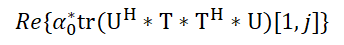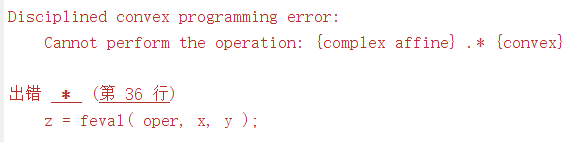# How to express this formula in CVX？

The specific formula is as follows:Where T is optimize variable, and T is an M*N matrix, U is a given matrix, alpha_0 is a complex number. Re is the operation which takes the real part of the complex number.

My MATLAB code is as follows:

The error reason isIt looks like a common mistake. So, how to solve this problem in CVX? I hope someone can provide an answer, I would greatly appreciate it！

I think
`real(alpha0'*[1,1i])*square_pos(norm(U'*T,'fro'))`
should work.

CVX doesn’t know how to deal with complex times convex (or concave), because that is neither convex nor concave. My rearrangement avoids that.

It really works. Thank you very much.